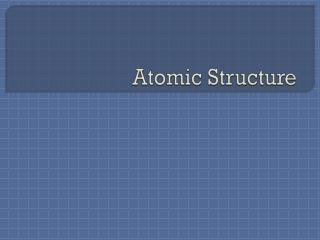DownloadDownload PresentationAtomic Structure

# Atomic Structure

Download Presentation## Atomic Structure

- - - - - - - - - - - - - - - - - - - - - - - - - - - E N D - - - - - - - - - - - - - - - - - - - - - - - - - - -
##### Presentation Transcript

1. Atomic Structure

2. Composition of the Atom There are three subatomic particles which make up the atom: Protons Electrons Neutrons

3. Protons • The proton is a positively charged particle which is found in the nucleus of the atom. • The number of protons of an element is also equal to the atomic number. • i.e. Sodium has an atomic number of 11 so therefore has 11 protons.

4. Neutrons • Neutrons are uncharged particles which are also found in the nucleus of the atom. • Neutrons are uncharged, but they do contain mass. • The number of neutrons can be found by subtracting the atomic number from the atomic mass.

5. i.e. Sodium contains 12 neutrons. 23- 11 = 12

6. Electrons Electrons are negatively charged particles which are found outside the nucleus of the atom in energy levels (orbitals)

7. In a neutral atom (meaning not charged), the number of protons is equal to the number of electrons. If an atom is positively charged this means that there are less electrons. In an negatively charged atom, there are more electrons.

8. i.e. Na+ has 11 protons, 12 neutrons and 10 electrons. The protons NEVER change, only the electrons change in charged atoms, AKA ions.

9. Try to find how many protons, electrons and neutrons are in the following atoms: O-2 Al+3

10. Isotopes While working with neon atoms, J.J. Thomson discovered two different types of neon atoms. Both were exactly the same chemically, but their masses were different.

11. Atoms of the same element but with different masses are known as isotopes. Since their masses differ, they have the same number of protons, but different numbers of neutrons.

12. For example, a neutral neon atom has 10 protons and 10 neutrons. Neon-21 isotope has 10 protons, but 11 neutrons. Neon-22 has 10 protons, and 12 neutrons

13. A particular type of atom containing a definite number of protons and neutrons is called a nuclide. • i.e. Protium , hydrogen-1, is a nuclide of hydrogen.

14. More sub-atomic Particles??? Protons and neutrons are not truly the smallest parts of matter. The electron however, as of present, is known to be an elementary particle.

15. The proton and neutron are composed of even smaller particles. • Scientists have divided sub-atomic particles into two broad categories: • Leptons • Hadrons

16. Leptons are true elementary particles. The electron is the most well-known lepton. Hadrons are made of even smaller particles. Protons and neutrons are examples are hadrons.

17. Hadrons are subdivided into mesons and baryons. Protons and neutrons are baryons. Baryons and mesons are made up of smaller particles called quarks.

18. Average Atomic Mass The atomic mass of an element is actually the average of all the isotopes’ masses. Not all the isotopes are present in equal amounts. Therefore when calculating the average atomic mass, we use a weighted average.

19. To find the average atomic mass: • First multiple each isotope by its abundance. • The products are then added together. • The sum is divided by the total abundance

20. Example In a sample of 100 neon atoms, 90 will be neon-20 and 10 will be neon-22. Calculate the average atomic mass of neon. Mass has units of atomic mass units (u) (90x19.992 u) + (10x21.991u)/100 = 20.192 u